AP State Syllabus AP Board 8th Class Maths Solutions Chapter 15 Playing with Numbers Ex 15.5 Textbook Questions and Answers.

## AP State Syllabus 8th Class Maths Solutions 15th Lesson Playing with Numbers Exercise 15.5Question 1.
Find the missing digits in the following additions.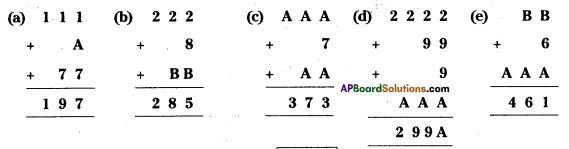Solution:
a) 1 + A + 7 = 17 ⇒ A = 17 – 8 = 9
∴ A = 9

b) 2 + 8 + B = 15 ⇒ B = 15 – 10 = 5
2 + 1 + B = 8 ⇒ B = 8 – 3 = 5
∴ B = 5

c) A + 7 + A=13 ⇒ 2A = 6 ⇒ A = 3 A + A + 1 = 7 2A = 6 ⇒ A = 3
∴ A = 3d) From 1st column
2 + 9 + 9 + A = 26
A = 26 – 20 = 6
From 2nd column
⇒ 2 + 1 + A = 9 ⇒ A = 9 – 3 = 6
∴ A = 6

e) B + 6 + A = 11 or 21
B + A + (1 or 2) = 6
A + 1 = 4 ⇒ A = 3
⇒ From (1), B + 6 + 3 = 11 ⇒ B = 2
∴ A = 3, B = 2

Question 2.
Find the value of A in the following
(a) 7A – 16 = A9 (b) 107 – A9 = lA (e) A36 – 1A4 = 742
Solution: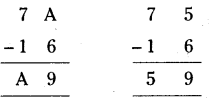a) 7A – 16 = A9
A- 6 = 9
If A = 5 it is possible.
∴ A = 5 (or)
7A- 16 = A9
⇒ 7 x 10 + (1 x A) – 16 = (A x 10 + 9 x 1)
⇒ 70 + A – 16 = 10A + 9
⇒ 9A = 45
⇒ A = 5b) 107 – A9 = 1A
⇒ 107 – (10 x A + 9 x 1) = (1 x 10 + A x 1)
⇒ 107-10A-9= 10 +A
⇒ 11A = 88 A = 8

c) A36 – 1A4 = 742
⇒ (100 x A + 3 x 10 + 6 x 1) – (1 x 100 + A x 10 + 4 x 1) = 742
⇒ 100A + 36 – 100 – 10A – 4 = 742
⇒ 90A = 810
⇒ A = $$\frac{810}{90}$$
∴ A = 9

Question 3.
Find the numerical value of the letters given below-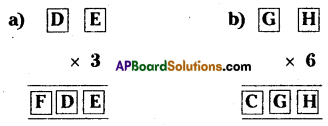Solution:
a) If E x 3 = E then E should be equal to 0 (or) 5.
5 x 3 = 15, 0 x 3 = 0
3 x D + 0 = ID [If E = 0]
⇒ 3D = 10 + D
⇒ 2D = 10
⇒ D = 5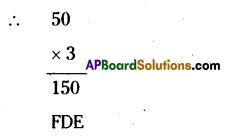∴ F = 1, D = 5, E = 0b) If H x 6 = H then H should be equal to 0, 2, 6, 8.
G6 = 1G [If H = 0]
⇒ 6G + 0 = 10 + G
⇒ 5G = 10
⇒ G = 10/5 = 2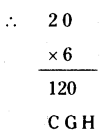C = 1, G = 2, H = 0

Question 4.
Replace the letters with appropriate digits
(a) 73K ÷ 8 = 9L
(b) 1MN ÷ 3 = MN
Solution:
a) 73K ÷ 8 = 9L
$$\frac{73 \mathrm{~K}}{8}$$ = 9L
If 73K is divisible by 8 then K = {1, 2, 3, …………. 9}
Select K = 6 from the set
∴ $$\frac{73 \mathrm{~K}}{8}$$ (R = 0)
∴ $$\frac{73 \mathrm{~K}}{8}$$ = 92 = 9L
⇒ 90 + 2 = (9 x 10 + L x 1)
⇒ 90 + 2 = 90 + L
∴ L = 2
∴ K = 6, L = 2b) 1MN ÷ 3 = MN
If 1MN is divisible by 3 then sum of all the digits is divisible by 3.
⇒ 1 + M + N = 3 x {1,2,3}
Let 1 + M + N = 3 x 2 = 6 say
M + N = 5 …………..(1)
$$\frac{1 \mathrm{MN}}{3}$$ = MN
⇒ 1MN = 3[MN]
⇒ 1 x 100 + M x 10 + N x 1
= 3[M x 10 + N x 1]
⇒ 100 + 10M + N = 3[10M + N]
⇒ 100 + 10M + N = 30M + 3N
⇒ 20M + 2N = 100
⇒ 10M + N = 50 …………….. (2)
From (1) & (2)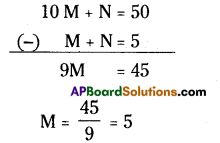10 M + N = 50 (-) M + N = 5 9M = 45
∴ M = 5
If M = 5 then
M + N = 5
⇒ N = 0
M = 5, N = 0 [∵ $$\frac{150}{3}$$ = 50 ]Question 5.
If ABB x 999 = ABC 123 (where A, B, C are digits) find the values of A, B, C.
Solution: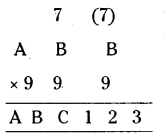From ABB x 999 = ABC 123, the product of units digit is equal to 3.
∴ B x 9 = 3 is the units digit.
If B = 7 then,
7 x 9 = 6 3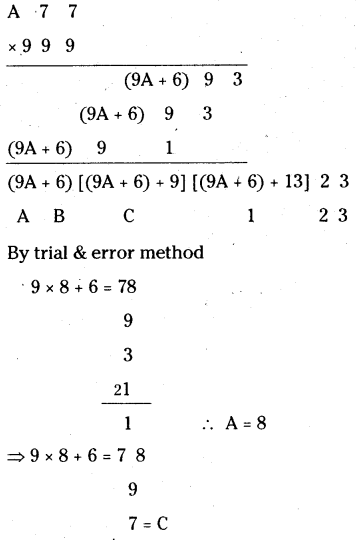∴ A = 8, B = 7, C = 6
∴ The required product = 876123
∴ A = 8, B = 7, C = 6